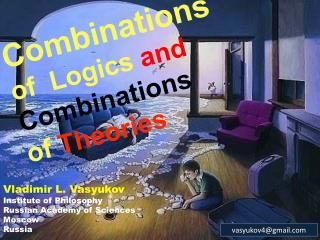DownloadDownload PresentationCombinations of Logics and Combinations of Theories

Combinations of Logics and Combinations of Theories

Télécharger la présentationCombinations of Logics and Combinations of Theories

- - - - - - - - - - - - - - - - - - - - - - - - - - - E N D - - - - - - - - - - - - - - - - - - - - - - - - - - -
Presentation Transcript

1. Combinations of Logics and Combinations of Theories Vladimir L. Vasyukov Institute of Philosophy Russian Academy of Sciences Moscow Russia vasyukov4@gmail.com

2. Abstract • Universal Logic would be treated as a general theory of logical systems consideredas a specific kind of mathematical structures the same manner Universal Algebratreats algebraic systems. • A category-theoretical approach where logicalsystems are combined in a category of the special sort provides us with some basisfor inquiring the Universe of Universal Logic. • In the framework of such an approachsome categorical constructions are introduced which describe the internal structure of the category of logical systems.

3. Signatures A signature is an indexed set = {n }nN, where each n is the setofn-aryconstructors. We consider that the set of propositional variables is included in 0.

4. Language The language over a given signature , which we denote by L, is built inductively in the usual way: • 0 L; • If nN, 1, . . . , nL and cn, then c(1, . . . , n)L . We call -formulas to the elements of L, or simply formulas when  is clear fromthecontext.

5. Logical Systems A logical system is a pair  = ,⊢, where  is a signature and ⊢ is a consequence operator on L (in the sense of Tarski), that is, ⊢: 2L 2L is a function that satisfies the following properties, for every , L : Extensiveness: ⊢; Monotonicity: If  then ⊢ ⊢; Idempotence: (⊢)⊢  ⊢ . Here ⊢ is a set of consequences of . For the sake of generality, we do not require that the consequence operator to be finitary, or even structural.

6. Combinations of Logics • Coproducts • would be characterized as • Σ1,1Σ2,2 = Σ1Σ2, 12 • where 12 is a consequence operator such that • Г iimpliesГ12 • forevery Г {}LΣi(i = 1, 2)

7. Combinations of Logics • Products • would be characterized as • Σ1, 1Σ2, 2 = Σ1Σ2, 12 • where 12 is a consequence operator such that • Г1,Г2 121,  2 • impliesГi iiforeveryГi{i} Σi • (i = 1, 2)

8. Combinations of Logics Coexponentials would be characterized as 21 = Σ1, 12 where 12 is a consequence operator such that Г12iffg[Г]2g() forall Log-morphismsg:12

9. Combinations of Logics • Exponentials • would be characterized as • 12 = Σ1, 12 • where12 is a consequence operatorsuch that • Г12iffthereexistLog-morphismsg:12 andh:21 such thath(g[Г])1h(g()).

10. Universe of Universal Logics A structure of the Universe of Universal Logics can be described as a threefold construction consisting of : A category Sig of signatures andtheirmorphisms(N-indexed families of functions h= {hn : 1n DC2n}nN, where DC k is the set of all derived connectives of arityk over  and a derived connective of aritykN is a -term d = 1. . .k. where Lk) C.Caleiro and R.Gonçalves

11. Universe of Universal Logics A category Log of logical systems and their morphisms(a signature morphismh : Σ1  Σ2 such that h[Φ1] h[Φ]2for every Φ LΣ1) C.Caleiro and R.Gonçalves

12. Universe of Universal Logics A category Tsp of theory spaces (complete lattices tsp = Th, where Th is the set of all theories of given logic) and their morphisms(functions h: Th1Th2 such that h(T) = h[T] for every TTh). C.Caleiro and R.Gonçalves

13. Universe of Universal Logics Equipollency Relation

14. Universe of Universal Logics Two formulas , are said to be logically equivalent in ,   , if both {}⊢ and  {}⊢ , or equivalently if {}⊢ = {}⊢. 1 = Σ1, 1 and 2 = Σ2, 2 are equipollent if and only if there exists Log-morphismsh: 12 and g: 21 such that the following conditions hold: •  1g(h()) for every L Σ1 ;  2h(g()) for every  LΣ2.

15. Universe of Universal Logics The equalityofLog-morphisms is the smallest equivalence relation betweenmorphisms such that • fg if and only if dom(f) is equipollent to dom(g) and codom(f) is equipollent tocodom(g), i.e. 1 and 2 are equipollenttogether with 1 and 2 are equipollent for logical system morphisms f : 12 , g : 12; • gf = h implies gfh; • ff and gg implies gfg f ; • f id1fid1f; • (hg)fh(gf) for all f,f: 12 , g,g: 23, h,h: 34.

16. Log is both a topos and a complement topos but all respective constructions works just up to the equivalence which is based exactly on the equipollence

17. Structure of Tsp • The last fact means that if we transfer categorical constructions from Logto Tsp then all equipollenceswill transform into usual categorical isomorphisms. • We obtain in Tspcoproducts, products, coexponentialsand exponentials with the respective diagrams. • All of them will be the complete lattices according to the properties of Th.

18. Structure of Tsp Tspis both a topos and a complement topos

19. The End

20. Thank you for your attention